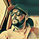# Williams Fractals [Tutorial/Template]

17499 vizualizações
17499
I noticed there weren't any code templates for Williams Fractals, therefore I have written this script to be a template and tutorial for those learning Pine Script. Each line is commented to show what it does.

If you don't know what Williams Fractals are, here is a brief explanation.

Bill Williams Fractals is a lagging indicator used to plot trend reversals on a chart. These appear over a candle at the point of reversal 2 candles after it has occurred. Often times this indicator is used in strategies such as Mumbai Scalping and paired with trend direction indicators and trailing stop indicators such as Parabolic SAR and SuperTrend.

If you like this script or use it's code in one of yours, please donate.
- PayPal: ma.spencer@gmx.com
- Bitcoin: 1foxypuyuoNp5n1LNCCCCmjZ4RAXntQ8X
```// Bill Williams Fractal Template
// Coded By: Matthew Spencer
// If you like this script or use it's code in one of yours, please donate.
// PayPal: ma.spencer@gmx.com Bitcoin: 1foxypuyuoNp5n1LNCCCCmjZ4RAXntQ8X

// Define our title and that this script will overlay the candles.
study("Williams Fractals",shorttitle="Fractals", overlay=true)

// Define "n" as the number of periods and keep a minimum value of 2 for error handling.
n = input(title="Periods", defval=2, minval=2, type=integer)

// Williams Fractals are a 5 point lagging indicator that will draw 2 candles behind.
// The purpose of the indicator is to plot points of trend reversals.
// Often these are paired with trailing stop indicators such as Parabolic SAR, Volatility Stop, and SuperTrend.

// Down pointing fractals occur over candles when:
//   High(n-2) < High(n)
//   High(n-1) < High(n)
//   High(n + 1) < High(n)
//   High(n + 2) < High(n)
dnFractal = (high[n-2] < high[n]) and (high[n-1] < high[n]) and (high[n+1] < high[n]) and (high[n+2] < high[n])

// Up pointing fractals occur under candles when:
//   Low(n-2) > Low(n)
//   Low(n-1) > Low(n)
//   Low(n + 1) > Low(n)
//   Low(n + 2) > Low(n)
upFractal = (low[n-2] > low[n]) and (low[n-1] > low[n]) and (low[n+1] > low[n]) and (low[n+2] > low[n])

// Plot the fractals as shapes on the chart.
plotshape(dnFractal, style=shape.arrowdown, location=location.abovebar, offset=-2, color=olive, transp=0) // Down Arrow above candles
plotshape(upFractal, style=shape.arrowup, location=location.belowbar, offset=-2, color=maroon, transp=0)  // Up Arrow below candles```You can use these for a 'fractal breakout' strategy. The idea is that fractals represent an inflection point in the market, a test of a price if you like, so breaking the fractal price level is considered a signal. Assuming we are going long, when the current price breaches the previous fractal high, wait until the *following* bar after the break and set a buy order just above the previous fractal high. Your stop goes at the lowest of the previous two fractal lows (or if the stock seems highly volatile you might just choose the previous fractal low). Unfortunately it doesn't work very well in the sample chart shown, in fact I think it is more relevant to stocks and commodities rather than forex trading (IIRC even Williams mentions that in his book).
RespostaI may have found a bug/edge-case. In this image you'll see your indicator in yellow and the default Williams Fractal in white. Notice how we're missing a fractal.

I was able to correct this by changing the comparison operator to `<=` in one case. Though, there are probably other edge cases unaccounted for. Too bad TradingView doesn't show us the Pine script for the built-in indicator.

Original:
dnFractal = (high < high) and (high < high) and (high < high) and (high < high)

Modified:
dnFractal = (high < high) and (high < high) and (high <= high) and (high < high)
Respostasiarian
@siarian, Are you sure you want 13 bars *after*? Seems like a very long time to wait for a signal to appear... even with fractals on the hour chart you can wait 2 frustrating hours for the fractal to appear (some people use a fractal variant of only one bar on each side to counter this)
Resposta